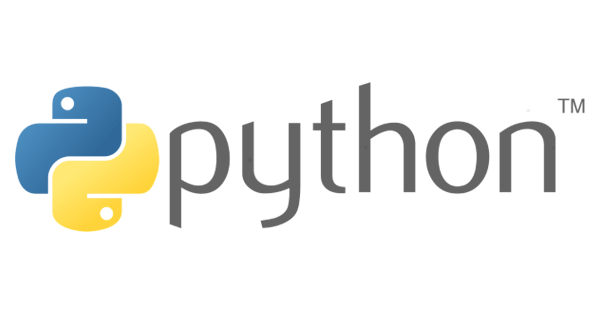# Python 資料型態## 查詢資料型態

``````print(type(100))
'''
<class 'int'>
'''

print(type('name'))
'''
<class 'str'>
'''``````

## Number (數值)

Python 3 有三種 Number 型態：

• int：整數。
• float：浮點數 (小數)。
• complex：複數。
``````print(type(5))
'''
<class 'int'>
'''

print(type(1.25))
'''
<class 'float'>
'''

print(type(3.14j))
'''
<class 'complex'>
'''``````

## String (字串)

String 使用成對 `'` 單引號或 `"` 雙引號來表示。

``````print('one')

print('two')

print('''one
two
three''')

print("""one
two
three""")``````

### 串接、重複字串

• 串接字串：使用 `+` 加號。
• 重複字串：使用 `*` 星號。
``````str = 'ABCDEF'

print(str + '-' + str)
'''
ABCDEF-ABCDEF
'''

print(str * 3)
'''
ABCDEFABCDEFABCDEF
'''``````

### 取得字串長度

``````print(len('ABCDEF'))
'''
6
'''``````

### 取值、切片

Python 的 String 是由字元序列所組成，它可以使用 `[]` 索引的方式來取值與切片。

Python 取值、切片參數表

`index` 0 -1
`start_index` 0 -1
`end_index` 0 -1
`start` 1 -1 1 不可為 0
 取值 前至後： 後至前： 切片 前至後： 後至前： 0 1 2 3 4 5 -6 -5 -4 -3 -2 -1 A B C D E F 0 1 2 3 4 5 -6 -5 -4 -3 -2 -1

``````str = 'ABCDEF'

print('''# 對照表

+---+---+---+---+---+---+
| A | B | C | D | E | F |
+---+---+---+---+---+---+

print('\n\n# [index] 取值')
print('str   ' + str)
print('str[-1]  ' + str[-1])        # 取得最後一個值

print('\n\n# [start_index:end_index] 切片')
print('str[2:5]     ' + str[2:5])
print('str[-2:]     ' + str[-2:])
print('str[-2:-5]   ' + str[-5:-2])

print('\n\n# [start_index:end_index:step] 切片')
print('str[::2]     ' + str[::2])   # 從前面開始，取得每 2 個間隔字元的字串
print('str[::-2]    ' + str[::-2])  # 從後面開始，取得每 2 個間隔字元的字串
print('str[::-1]    ' + str[::-1])  # 從後面開始，取得每 1 個間隔字元的字串 (就是將字串反轉)

'''
# 對照表

+---+---+---+---+---+---+
| A | B | C | D | E | F |
+---+---+---+---+---+---+

# [index] 取值
str   A
str[-1]  F

# [start_index:end_index] 切片
str[2:5]     CDE
str[-2:]     EF
str[-2:-5]   BCD

# [start_index:end_index:step] 切片
str[::2]     ACE
str[::-2]    FDB
str[::-1]    FEDCBA
'''``````

## List (串列)

• 具有索引。
• 長度可變動。
Python List 常用函式

`list(s)` 元素數量 List 型態 返回元素數量
`max(iterable)` 元素最大值 List 型態 返回元素的最大值
`min(iterable)` 元素最小值 List 型態 返回元素的最小值
Python List 常用方法

`list.append(x)`   元素 在未尾增加一個元素 更動 List
`list.extend(L)`   List 型態 在未尾增加一個 List 型態
`list.insert(i, x)`   `i` 欲插入的 index 位置 `x` 插入的元素 插入元素至指定位置
`list.pop(i = -1)` 移除的元素 index 移除元素
`list.remove(x)`   元素值 移除元素 更動 List

``````# 建立一個空 List
list = []

# 查看資料型態
print(type(list))
'''
<class 'list'>
'''

# 無法這樣新增元素，但如果該 index 已設值，可這樣更改值
# list = 1
'''
Traceback (most recent call last):
File "<stdin>", line 1, in <module>
IndexError: list assignment index out of range
'''

# 在未尾增加一個元素
list.append('one')
print(list)
'''
['one']
'''

# 更改第 1 (啟始值0) 個元素的值
list = 1
print(list)
'''

'''

# List 可存放各種元素
list = [1, 'one', True]
print(list)
'''
[1, 'one', True]
'''

# 查看 List 有多少元素
print(len(list))
'''
3
'''

# 也可以使用字串取值與切片的方式
listStr = ['A', 'B', 'C', 'D', 'E', 'F']
listNum = [1, 2, 3, 4, 5, 6]
print(listStr)
print(listStr[1:3])
'''
A
['B', 'C']
'''

# 使用 * 符號重複操作
print(listStr * 5)
'''
AAAAA
'''

# 使用 + 符號串接兩個 List
print(listStr + listNum)
'''
['A', 'B', 'C', 'D', 'E', 'F', 1, 2, 3, 4, 5, 6]
'''

# 使用 del 來刪除元素或 List
del listStr
print(listStr)
del listStr
'''
['B', 'C', 'D', 'E', 'F']
'''``````

## Tuple (元組)

Tuple 與 List 類似，使用方法可直接參考 List，除下述不同：

• 使用 `()` 括號建立 Tuple。

## Set (集合)

Python Set 運算表

| 聯集 'A', B', 'C' 1, 2, 'A' 'A', 'B', 'C', 1, 2 合併 set1 與 set2 不重複的值
& 交集 'A' set1 與 set2 相同的值
- 差集 'B', 'C' 在 set1 但不存在 set2 的值
^ XOR 'B', 'C', 1, 2 排除相同的值

``````set1 = {'A', 'B', 'C'}
set2 = {1, 2, 'A'}

# 測試元素是否在 Set 中
print('A' in set1)
'''
True
'''

# 聯集
print(set1 | set2)
'''
{1, 2, 'B', 'A', 'C'}
'''

# 交集
print(set1 & set2)
'''
{'A'}
'''

# 差集
print(set1 - set2)
'''
{'C', 'B'}
'''

# XOR
print(set1 ^ set2)
'''
{1, 2, 'C', 'B'}
'''``````

## Dictionary (字典)

``````dict = {'name': 'Jacky', 'sex': 'F', 'height': 172, 'weight': 52}

print(dict)
print(dict['name'])
'''
{'weight': 52, 'sex': 'F', 'name': 'Jacky', 'height': 172}
Jacky
'''``````